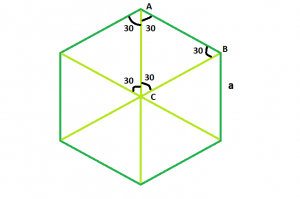# Find length of Diagonal of Hexagon

Given a regular hexagon of side length a, the task is to find the length of it’s diagonal.

Examples:

```Input : a = 4
Output : 8

Input : a = 7
Output : 14
```## Recommended: Please try your approach on {IDE} first, before moving on to the solution.

From the diagram, it is clear that the triangle ABC is an equilateral triangle, so
AB = AC = BC = a.

also it is obvious, diagonal = 2*AC or 2*BC
So the length of diagonal of the hexagon = 2*a

Below is the implementation of the above approach:

## C++

 `// C++ Program to find the diagonal ` `// of the hexagon ` `#include ` `using` `namespace` `std; ` ` `  `// Function to find the diagonal ` `// of the hexagon ` `float` `hexadiagonal(``float` `a) ` `{ ` `    ``// side cannot be negative ` `    ``if` `(a < 0) ` `        ``return` `-1; ` ` `  `    ``// diagonal of the hexagon ` `    ``return` `2 * a; ` `} ` ` `  `// Driver code ` `int` `main() ` `{ ` `    ``float` `a = 4; ` ` `  `    ``cout << hexadiagonal(a) << endl; ` ` `  `    ``return` `0; ` `} `

## Java

 `// Java Program to find the diagonal ` `// of the hexagon ` ` `  `import` `java.io.*; ` ` `  `class` `GFG { ` ` `  `// Function to find the diagonal ` `// of the hexagon ` `static` `float` `hexadiagonal(``float` `a) ` `{ ` `    ``// side cannot be negative ` `    ``if` `(a < ``0``) ` `        ``return` `-``1``; ` ` `  `    ``// diagonal of the hexagon ` `    ``return` `2` `* a; ` `} ` ` `  `// Driver code ` ` `  ` `  `    ``public` `static` `void` `main (String[] args) { ` `            ``float` `a = ``4``; ` ` `  `    ``System.out.print( hexadiagonal(a)); ` `} ` `} ` ` `  `// This code is contributed ` `// by shs `

## Python3

 `# Python3 Program to find the diagonal ` `# of the hexagon ` ` `  `# Function to find the diagonal ` `# of the hexagon ` `def` `hexadiagonal(a): ` ` `  `    ``# side cannot be negative ` `    ``if` `(a < ``0``): ` `        ``return` `-``1` ` `  `    ``# diagonal of the hexagon ` `    ``return` `2` `*` `a ` ` `  ` `  `# Driver code ` `if` `__name__``=``=``'__main__'``: ` `    ``a ``=` `4` `    ``print``(hexadiagonal(a)) ` ` `  `# This code is contributed by  ` `# Shivi_Aggarwal `

## C#

 `// C# Program to find the diagonal ` `// of the hexagon ` `using` `System; ` ` `  `class` `GFG { ` ` `  `    ``// Function to find the diagonal ` `    ``// of the hexagon ` `    ``static` `float` `hexadiagonal(``float` `a) ` `    ``{ ` `        ``// side cannot be negative ` `        ``if` `(a < 0) ` `            ``return` `-1; ` `         `  `        ``// diagonal of the hexagon ` `        ``return` `2 * a; ` `    ``} ` ` `  `// Driver code ` `public` `static` `void` `Main() ` `{ ` `   ``float` `a = 4; ` `   ``Console.WriteLine( hexadiagonal(a)); ` `} ` `} ` ` `  `// This code is contributed ` `// by anuj_67.. `

## PHP

 ` `

Output:

```8
```

Attention reader! Don’t stop learning now. Get hold of all the important DSA concepts with the DSA Self Paced Course at a student-friendly price and become industry ready.

My Personal Notes arrow_drop_upCheck out this Author's contributed articles.

If you like GeeksforGeeks and would like to contribute, you can also write an article using contribute.geeksforgeeks.org or mail your article to contribute@geeksforgeeks.org. See your article appearing on the GeeksforGeeks main page and help other Geeks.

Please Improve this article if you find anything incorrect by clicking on the "Improve Article" button below.